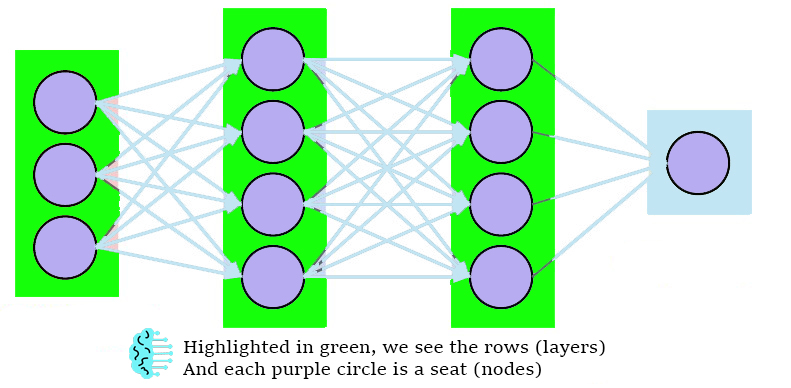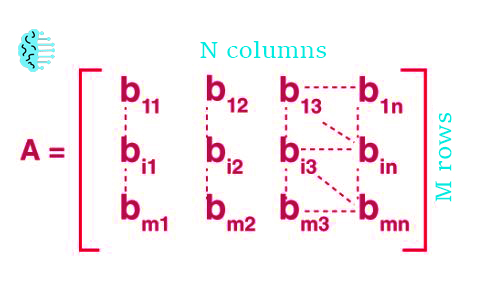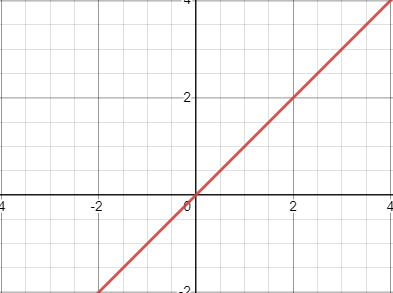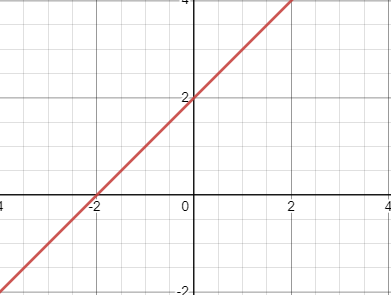# Dense Layer: The Building Block to Neural Networks

The Dense layer is a critical component in Machine Learning.

While the most straightforward layer, the dense layer is still vital in any neural network design and is one of the most commonly used layers.

Below we will be breaking down the output generated from a dense layer, input arrays, and the difference between a dense layer versus some other layers.

## What is a layer?

Layers are made of nodes, and the nodes provide an environment to perform computations on data.

In simpler terms, think of a neural network as a stadium, a layer as a row of seats in a stadium, and a node as each seat.

A node combines the inputs of a data set with a weighted coefficient which either increases or dampens inputs.These rows and seats work together to get us to the final output layer, which will contain our final answers (based on how we defined the previous layer)

### What is Keras?

Keras is a Python API that runs on top of the Machine Learning Platform Tensorflow.

Keras enables users to add several prebuilt layers in different Neural network architectures.

When TensorFlow was initially released, it was pretty challenging to use.

Learning any Machine Learning framework will not be easy, and there will always be a learning curve, but early TensorFlow was pretty low-level and took a ton of time to learn.

Keras is a python library that builds on the top of TensorFlow, which has a user-friendly interface, faster production deployment, and faster initial development of machine learning models.

Using Keras makes the overall experience of TensorFlow easier.

Realize, before 2017, Keras was only a stand-alone API.

Now, TensorFlow has fully integrated Keras, but you can still use the Keras API by itself, and the stand-alone API usually is more up-to-date with newer features.

Understanding features during modeling is important. We wrote Keras Feature Importance to give a good intro so you could understand your models better.

## Keras Layers

Keras Layers are the building blocks of the whole API.

We will stack these layers together to create our models, but you could also have a single dense layer that acts as something as simple as a linear regression model or multiple dense layers (with a hidden layer) to create a neural network.

Changing one of the layers in a neural network will change the results in the final output arrays.

### Types of Layers in Keras

The core layers within the Keras API are

• Dense Layer
• Input Layer
• Activation Layer
• Embedding Layer
• Lambda Layer

The Dense Layer is the most commonly used, and there is some slight overlap in these Keras layers.

For example, a parameter passed within a dense layer can be the activation function, or you can pass an activation function as a layer in a sequential model.

In future posts, we will be going more in-depth into activation functions and other deep learning model features. More information on modeling can be found here at steps per epoch keras.

## What the Dense Layer Performs

The dense layer performs the following calculation

outputs = activation(dot(input, kernel) + bias)

Let’s break this down a bit (from the inside out).

### What is the Input Matrix

Your input data passed will be as a matrix into your dense layer.

If your input data, for example, was a data frame with m rows and n columns.

Your matrix will be the same m rows and n columns, just lacking column identifiers.We go over input data in-depth and much more about Keras in our other post, Keras Shuffle.

### What is the Kernel Weights Matrix

Each Kernel weight matrix is specific to that dense layer and node (think about row number and seat number).

The kernel weights matrix is the heart of the neural network; as the data progresses from dense to dense layers, these weights will be updated based on backpropagation. (Learn more)

The Kernel weights matrix is updated after every run, and the new weights matrix created will contain new weights to multiply the input data by.

The weight matrix is crucial to understand. Many newcomers to machine learning have trouble understanding the vector shape needed to do the dot product between the input data and weight matrix.

### What is the Dot Product of the Input and Kernel?

The output size of the dot product between the input and kernel will be a single scalar value.

This throws some people off who are expecting another matrix from the dot product and are unfamiliar with the differences. (See the difference).

The value received from this dot product of the Input and Kernel is the value that will be passed onward in your neural network before applying any bias to it.

### What is the Bias Vector?

To understand the bias vector, let’s go back to one of the most simple fundamentals of mathematics.

#### The equation of a line

y = mx + B

Now, I know it isn’t talked about a bunch, but that B term is the bias of a line.

Understanding bias’s effect is simpler when you can see it in action.

##### Here is the equation y = 1x + 0##### Here is the equation y= 1x + 2In our first picture, even though the line is the same, our line never went through (2,2), and if our function we’re trying to predict value (2,2), it wouldn’t be possible.

However, once we added bias, our function went right through the point (2,2) and would give us that exact prediction with input x = 2.

Now, a bias vector is this same logic; just instead of one vector term, there is n number of vector terms, where n is the size of your vector.

### Keras Dense Layer Activation Function

Here is a list of the different dense layer activation functions

• relu
• sigmoid
• softmax
• softplus
• softsign
• tanh
• selu
• elu
• exponential

### Activation function

We know how the inside of our dense layer formula works; the last part is the activation function.

Remember, after our bias is applied, we will have a vector.

So, we have

outputs = activation(vector)

Where our activation can be anything chosen above, we will select the relu activation function for this.

The relu function will take each value in the vector and keep it if it’s above zero or replace it with zero if not.

#### Dense Layer Examples

For example, input vector = [-1,2,-4,2,4] (after out dot product and applying our bias vector)

Starting your model correctly is how you have success during modeling, we will teach you how in Keras Input Shape.

Will become output vector = [0,2,0,2,4] with the same output shape.